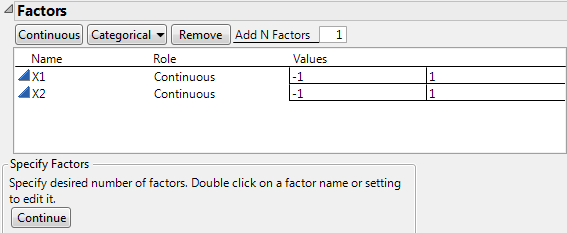Publication date: 06/16/2020

## Factors

Add factors in the Factors outline.

Figure 21.4 Factors OutlineThe Factors outline contains these options:

Continuous

Enters the number of continuous factors specified in Add N Factors.

Categorical

Enters the number of nominal factors specified in Add N Factors.

Remove

Removes the selected factors.

Adds multiple factors of a given type. Enter the number of factors to add and click Continuous or Categorical. Repeat Add N Factors to add multiple factors of different types.

Tip: When you have completed your Factors panel, select Save Factors from the red triangle menu. This saves the factor names and values in a data table that you can later reload. See Space Filling Design Options.

### Factors Outline

The Factors outline contains the following columns:

Name

The name of the factor. When added, a factor is given a default name of X1, X2, and so on. To change this name, double-click it and enter the desired name.

Role

Specifies the Design Role of the factor. The Design Role column property for the factor is saved to the data table. This property ensures that the factor type is modeled appropriately.

Values

The experimental settings for the factors. To insert Values, click the default values and enter the desired values.

#### Editing the Factors Outline

In the Factors outline, note the following:

To edit a factor name, double-click the factor name.

Categorical factors have a down arrow to the left of the factor name. Click the arrow to add a level.

To remove a factor level, click the value, click Delete, and click outside the text box.

To edit a value, click the value in the Values column.

### Factor Types

Continuous

Numeric data types only. A continuous factor is a factor that you can conceptually set to any value between the lower and upper limits you supply, given the limitations of your process and measurement system.

Categorical

Either numeric or character data types. For a categorical factor, the value ordering is the order of the values as entered from left to right. This ordering is saved in a Value Order column property after the design data table is created.

### Factor Column Properties

For each factor, various column properties are saved to the data table.

Design Role

Each factor is assigned the Design Role column property. The Role that you specify in defining the factor determines the value of its Design Role column property. The Design Role property reflects how the factor is intended to be used in modeling the experimental data. Design Role values are used in the Augment Design platform.

Factor Changes

Each factor is assigned the Factor Changes column property with a setting of Easy. In space-filling designs, it is assumed that factor levels can be changed for each experimental run. Factor Changes values are used in the Evaluate Design and Augment Design platforms.

Coding

If the Role is Continuous, the Coding column property for the factor is saved. This property transforms the factor values so that the low and high values correspond to –1 and +1, respectively. The estimates and tests in the Fit Least Squares report are based on the transformed values.

Value Order

If the Role is Categorical or Blocking, the Value Order column property for the factor is saved. This property determines the order in which levels of the factor appear.